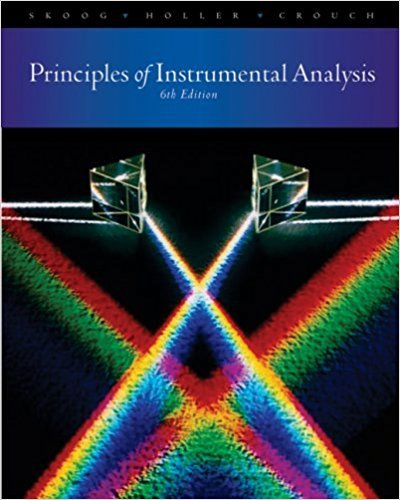×
×

# Quantitative X-ray photoelectron spectrometry has become more popular inrecent yearsISBN: 9780495012016 317

## Solution for problem 21-14 Chapter 21

Principles of Instrumental Analysis | 6th Edition

• Textbook Solutions
• 2901 Step-by-step solutions solved by professors and subject experts
• Get 24/7 help from StudySoup virtual teaching assistantsPrinciples of Instrumental Analysis | 6th Edition

4 5 1 410 Reviews
12
5
Problem 21-14

Quantitative X-ray photoelectron spectrometry has become more popular inrecent years. The factors relating the intensity of emission to atomic concentration(density) are given in Equation 21-3.(a) Of the factors influencing the emission intensity, identify those related to theanalyte.(b) Identify the factors influencing the emission intensity related to the spectrometer.(c) The measured quantity in quantitative XPS is usually liS, where I is the peakarea and S is the sensitivity factor for the element of interest. If this quantityis measured for the analyte (liS), and the corresponding value measured foran internal standard with a different transition (liS)" show by means of anequation how the ratio (/S),/(/S), is related to the atomic concentrationratio of the analyte to the internal standard.(d) If all the elements on a surface arc measured by XPS, show that the fractionalatomic concentration fA of element A is given by where In is the measured peak area for element n, Sn is the atomic sensitivityfactor for that peak, and the summation is carried out over all n.(e) For a polyurethane samplc in which carbon, nitrogen, and oxygen were detectedby XPS, the sensitivity factor for C was 0.25 on the spectrometerused. The sensitivity factor for N was 0.42 and that for 0 was 0.66 on thesame spectrometer. If the peak areas were C(ls) = 26,550, N(Is) = 4475,and O( Is) = 13,222, what were the atomic concentrations of the threeelements')(f) What are the limitations of quantitative analysis with XPS" Why might theatomic concentrations measured not correspond to the bulk composition"(g) For polyurethane, the stoichiometric atomic concentrations are C = 76.0%,N = 8.0%, and 0 = 16.0%. Calculate the percentage error in the valuesobtained in part (e) for each element.

Step-by-Step Solution:
Step 1 of 3

Friday, September 2, 2016 Chapter 2: Atoms and Elements Law of Conservation of Mass -Lavoisier - In a chemical reaction, Matter is nighter created nor destroyed - the total mass of the materials you have before the reaction must equal the total mass of the materials you have after the reaction - Total mass of reactants = Total mass...

Step 2 of 3

Step 3 of 3

##### ISBN: 9780495012016

This full solution covers the following key subjects: . This expansive textbook survival guide covers 34 chapters, and 619 solutions. The answer to “Quantitative X-ray photoelectron spectrometry has become more popular inrecent years. The factors relating the intensity of emission to atomic concentration(density) are given in Equation 21-3.(a) Of the factors influencing the emission intensity, identify those related to theanalyte.(b) Identify the factors influencing the emission intensity related to the spectrometer.(c) The measured quantity in quantitative XPS is usually liS, where I is the peakarea and S is the sensitivity factor for the element of interest. If this quantityis measured for the analyte (liS), and the corresponding value measured foran internal standard with a different transition (liS)" show by means of anequation how the ratio (/S),/(/S), is related to the atomic concentrationratio of the analyte to the internal standard.(d) If all the elements on a surface arc measured by XPS, show that the fractionalatomic concentration fA of element A is given by where In is the measured peak area for element n, Sn is the atomic sensitivityfactor for that peak, and the summation is carried out over all n.(e) For a polyurethane samplc in which carbon, nitrogen, and oxygen were detectedby XPS, the sensitivity factor for C was 0.25 on the spectrometerused. The sensitivity factor for N was 0.42 and that for 0 was 0.66 on thesame spectrometer. If the peak areas were C(ls) = 26,550, N(Is) = 4475,and O( Is) = 13,222, what were the atomic concentrations of the threeelements')(f) What are the limitations of quantitative analysis with XPS" Why might theatomic concentrations measured not correspond to the bulk composition"(g) For polyurethane, the stoichiometric atomic concentrations are C = 76.0%,N = 8.0%, and 0 = 16.0%. Calculate the percentage error in the valuesobtained in part (e) for each element.” is broken down into a number of easy to follow steps, and 277 words. The full step-by-step solution to problem: 21-14 from chapter: 21 was answered by , our top Chemistry solution expert on 03/02/18, 06:21PM. Since the solution to 21-14 from 21 chapter was answered, more than 216 students have viewed the full step-by-step answer. This textbook survival guide was created for the textbook: Principles of Instrumental Analysis , edition: 6. Principles of Instrumental Analysis was written by and is associated to the ISBN: 9780495012016.

Unlock Textbook Solution